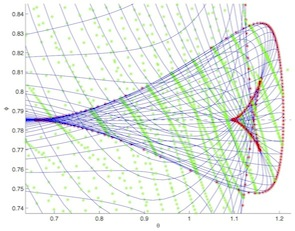# Logiciels

Cette rubrique recense plusieurs contributions de certains membres du groupe dans le développement logiciel autour de l’optimisation et du contrôle optimal.Version 10 of INTLAB :

Version 10 of INTLAB, the Matlab/Octave toolbox for Reliable Computing, is now available. Several functions of global nature have been added, in particular :Global minimizationGlobal constraint minimizationAll roots of a nonlinear system R^n->R^nAll roots of the gradient of a nonlinear system R^n->RParameter identification
Results computed by INTLAB are verified to be correct in a rigorous mathematical sense. That includes all possible computational inaccuracies, from the input data to the output. In particalar, input data may be afflicted with tolerances. In that case error bounds for the solution of all problems for data within the tolerances are computed. Several other routines have been improved, in particular the sparse linear system solver. For more information click here. INTLAB is entirely written in Matlab/Octave, running on the latest Matlab 2017a and downward compatible.Optimisation stochastique :

New stochastic optimization library. The StOpt library developped at OSIRIS department at EDF R&D and used in some operational projects is released as an open source project.

The STochastic OPTimization library (StOpt) aims at providing tools in C++ for solving some stochastic optimization problems encountered in finance or in the industry. A python binding is available for some C++ objects provided permitting to easily solve an optimization problem by regression.

Different methods are available :Dynamic programming methods based on Monte Carlo with regressions (global, local and sparse regressors), for underlying states following some uncontrolled Stochastic Differential Equations (python binding provided).Semi-Lagrangian methods for Hamilton Jacobi Bellman general equations for underlying states following some controlled Stochastic Differential Equations (C++ only)Stochastic Dual Dynamic Programming methods to deal with stochastic stocks management problems in high dimension (C++ only)
For each method, a framework is provided to optimize the problem and then simulate it out of the sample using the optimal commands previously calculated. Parallelization methods based on OpenMP and MPI are provided in this framework permitting to solve high dimensional problems on clusters.Contrôle optimal :Optimisation globale :

Exemple de simulation numérique avec le problème du micro-nageur : Controllability and Optimal Strokes for N-link Microswimmer